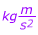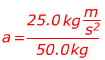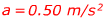# Force Formula

Force Formula

Force is the mass of an object, multiplied by its acceleration. The unit of force is. This is called a Newton, with the symbol N. Force has a magnitude and a direction.

force = mass x acceleration

F = ma

F = force

m = mass

a = acceleration

Force Formula Questions:

1) A 0.15 kg coconut falls out of its tree. The acceleration due to gravity is 9.80 m/s2, down. What is the force acting on the coconut due to gravity?

Answer: The force can be found using the equation:

F = ma

F = (0.15 kg)(9.80 m/s2)

F = 1.47F = 1.47 N

The force due to gravity acting on the coconut is 1.47 N, down.

2) A man pushes a 50.0 kg block of ice across a frozen pond. He applies a force of 25.0 N, pushing the block away from him. What is the acceleration of the block?

Answer: To find the acceleration, rearrange the equation:The block is accelerating 0.50 m/s2, directed away from the man.

 Related Links: Force, Mass, Acceleration Quiz Force and Acceleration Quiz Mass Examples Gravity Examples